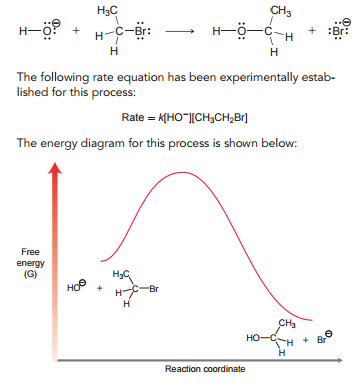# Problem: Consider the following reaction: (g) Draw the transition state of this process, and identify its location on the energy diagram.

###### Problem Details

Consider the following reaction:

(g) Draw the transition state of this process, and identify its location on the energy diagram.What scientific concept do you need to know in order to solve this problem?

Our tutors have indicated that to solve this problem you will need to apply the Hammond Postulate concept. You can view video lessons to learn Hammond Postulate. Or if you need more Hammond Postulate practice, you can also practice Hammond Postulate practice problems.

What is the difficulty of this problem?

Our tutors rated the difficulty ofConsider the following reaction: (g) Draw the transition st...as medium difficulty.

How long does this problem take to solve?

Our expert Organic tutor, Chris took 1 minute and 38 seconds to solve this problem. You can follow their steps in the video explanation above.

What textbook is this problem found in?

Our data indicates that this problem or a close variation was asked in Organic Chemistry - Klein 1st Edition. You can also practice Organic Chemistry - Klein 1st Edition practice problems.